# Newtons 3 rd Law Action and Reaction Force

• Slides: 11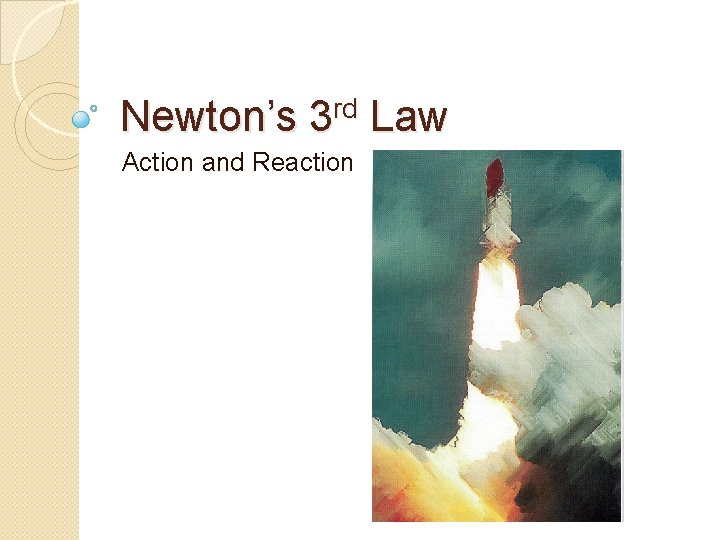Newton’s 3 rd Law Action and ReactionForce Pairs �A force is always applied by a secondary object �The object to which the force is applied also exerts a force back on the initial object �Whenever there is one force, there is a second force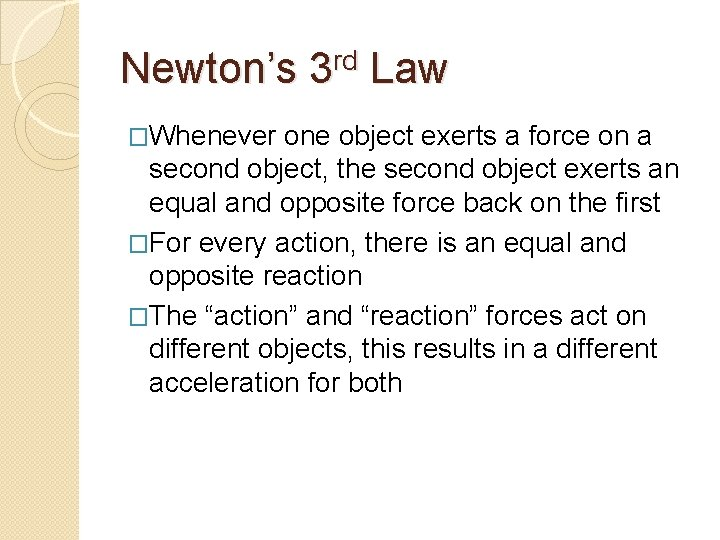Newton’s 3 rd Law �Whenever one object exerts a force on a second object, the second object exerts an equal and opposite force back on the first �For every action, there is an equal and opposite reaction �The “action” and “reaction” forces act on different objects, this results in a different acceleration for both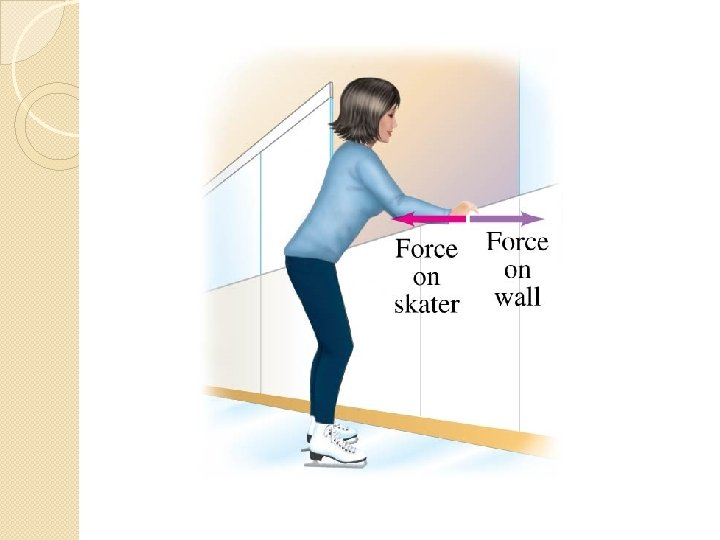Example �Two carts, one with a mass of 3 kg, and the other with a mass of 1 kg are sitting end to end on a desk. One the “explodes” and exerts a force of 50 N on the other, what is the acceleration of each?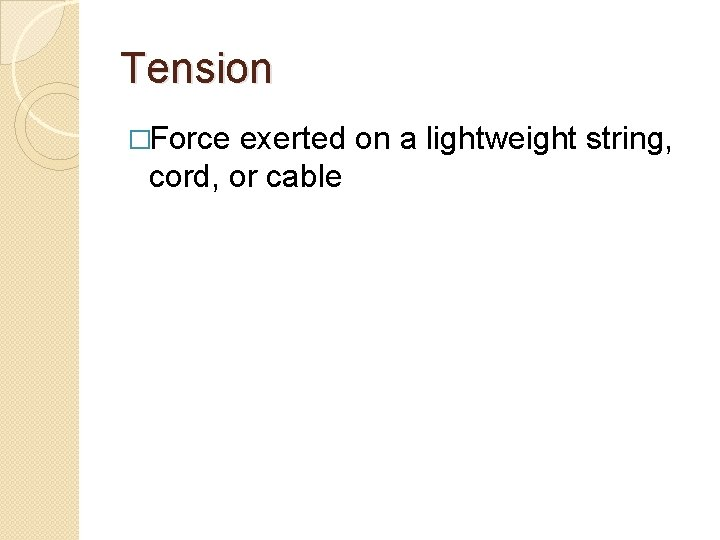Tension �Force exerted on a lightweight string, cord, or cableExample �Two blocks are attached by a string; block A is on the left and has a mass of 20 kg, block B on the right and has a mass of 10 kg. Block A is pulled with a force of 60 N (W). Find the acceleration of the two blocks and the tension on the string between the two.Example An elevator uses a counterweight as shown. The mass of the counterweight is 1000 kg and the mass of a loaded elevator is 1150 kg. Calculate the acceleration of the elevator and the tension in the cable.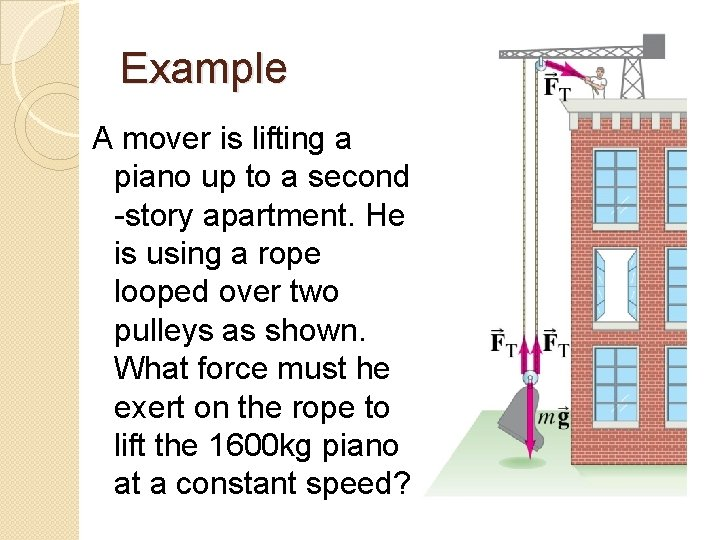Example A mover is lifting a piano up to a second -story apartment. He is using a rope looped over two pulleys as shown. What force must he exert on the rope to lift the 1600 kg piano at a constant speed?Example A small 50 g mass hangs from a thin string in a car and can swing like a pendulum as shown. What is the angle and force of tension on the string when the car a) accelerates forward at 1. 20 m/s 2 b) moves at a constant velocity of 90 km/hr